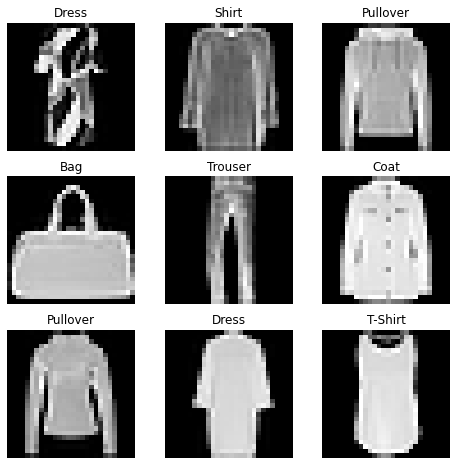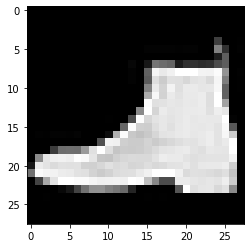The behavior of OneFlow's Dataset and DataLoader is the same as PyTorch. Both Dataset and DataLoader are designed for making dataset management decoupling with model training.

Dataset classes are used to define how to read data. For common computer vision datasets (e.g. FashionMNIST), we can use the dataset classes from datasets module of FlowVision library. These dataset classes can help us download and load some prevailing datasets automatically, and all of them inherit the Dataset class indirectly. For other datasets, we can define custom dataset classes through inheriting the Dataset class.

DataLoader wraps Dataset into an iterator, for easy iterating and access to samples during training.

import matplotlib.pyplot as plt

import oneflow as flow
import oneflow.nn as nn
from oneflow.utils.data import Dataset
from flowvision import datasets
from flowvision import transforms

The flowvision.transforms imported above provides some image data transformation operations (e.g. ToTensor is able to convert PIL images or NumPy ndarrays to tensors), which can be used in dataset classes directly.

Here is an example of how to load FashionMNIST dataset by flowvision.datasets.

We pass the following parameters to the FashionMNIST class: - root: the path where the train/test data is stored; - train: True for training dataset, False for test dataset; - download=True: downloads the data from the internet if it’s not available at root; - transforms: the feature and label transformations.

training_data = datasets.FashionMNIST(
root="data",
train=True,
transform=transforms.ToTensor(),
source_url="https://oneflow-public.oss-cn-beijing.aliyuncs.com/datasets/mnist/Fashion-MNIST/",
)

test_data = datasets.FashionMNIST(
root="data",
train=False,
transform=transforms.ToTensor(),
source_url="https://oneflow-public.oss-cn-beijing.aliyuncs.com/datasets/mnist/Fashion-MNIST/",
)


The first time it runs, it will download the data set and output the following:

Downloading https://oneflow-public.oss-cn-beijing.aliyuncs.com/datasets/mnist/Fashion-MNIST/train-images-idx3-ubyte.gz
26422272/? [00:02<00:00, 8090800.72it/s]
Extracting data/FashionMNIST/raw/train-images-idx3-ubyte.gz to data/FashionMNIST/raw

29696/? [00:00<00:00, 806948.09it/s]
Extracting data/FashionMNIST/raw/train-labels-idx1-ubyte.gz to data/FashionMNIST/raw

4422656/? [00:00<00:00, 19237994.98it/s]
Extracting data/FashionMNIST/raw/t10k-images-idx3-ubyte.gz to data/FashionMNIST/raw

6144/? [00:00<00:00, 152710.85it/s]
Extracting data/FashionMNIST/raw/t10k-labels-idx1-ubyte.gz to data/FashionMNIST/raw


## Iterating the Dataset¶

We can index Dataset manually like a list: training_data[index]. The following example randomly accesses 9 pictures in training_data and visualizes them.

labels_map = {
0: "T-Shirt",
1: "Trouser",
2: "Pullover",
3: "Dress",
4: "Coat",
5: "Sandal",
6: "Shirt",
7: "Sneaker",
8: "Bag",
9: "Ankle Boot",
}
figure = plt.figure(figsize=(8, 8))
cols, rows = 3, 3
from random import randint
for i in range(1, cols * rows + 1):
sample_idx = randint(0, len(training_data))
img, label = training_data[sample_idx]
plt.title(labels_map[label])
plt.axis("off")
plt.imshow(img.squeeze().numpy(), cmap="gray")
plt.show()## Creating a Custom Dataset for Your Files¶

A custom dataset can be defined by inheriting oneflow.utils.data.Dataset. Custom Dataset can be used with Dataloader introduced in the next section to simplify data processing.

Here is an example of how to create a custom Dataset, the key steps are:

• Inheriting oneflow.utils.data.Dataset
• Implements the __len__ method that returns the number of samples in our dataset.
• Implements the __getitem__ method that loads and returns a sample from the dataset when users call dataset_obj[idx].
import numpy as np
class CustomDataset(Dataset):
raw_data_x = np.array([[1, 2], [2, 3], [4, 6], [3, 1]], dtype=np.float32)
raw_label = np.array([, , , ], dtype=np.float32)

def __init__(self, transform=None, target_transform=None):
self.transform = transform
self.target_transform = target_transform

def __len__(self):
return len(raw_label)

def __getitem__(self, idx):
x = CustomDataset.raw_data_x[idx]
label = CustomDataset.raw_label[idx]
if self.transform:
x = self.transform(x)
if self.target_transform:
label = self.target_transform(label)
return x, label

custom_dataset = CustomDataset()
print(custom_dataset)
print(custom_dataset)


Output：

(array([1., 2.], dtype=float32), array([8.], dtype=float32))
(array([2., 3.], dtype=float32), array([13.], dtype=float32))


The Dataset retrieves all features of our dataset and labels one sample at a time. While training a model, we typically want to pass samples in "minibatches", which means they will load a same amount of data as the batch size at the time, and reshuffle the data at every epoch to reduce model overfitting.

At this time, we can use DataLoader. DataLoader can wrap Dataset into an iterator to access data during the training loop. Here is an example:

• batch_size=64: the batch size at each iteration
• shuffle: whether the data is shuffled after we iterate over all batches
from oneflow.utils.data import DataLoader

print(f"shape of x:{x.shape}, shape of label: {label.shape}")


Output：

shape of x:oneflow.Size([64, 1, 28, 28]), shape of label: oneflow.Size()


img = x.squeeze().numpy()
label = label
plt.imshow(img, cmap="gray")
plt.show()
print(label)


Output：(output a picture randomly)tensor(9, dtype=oneflow.int64)


We can also use the DataLoader iterator during the training loop.

for x, label in train_dataloader:
print(x.shape, label.shape)
# training...